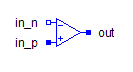Multiphase Closing Switch with Arc - MapleSim Help

Multiphase Closing Switch With Arc

Multiphase closer with arcDescription The Multiphase Closing Switch with Arc (or Closer With Arc) component contain $m$ single-phase closing switch with arc models, each connected between corresponding phases of ${\mathrm{plug}}_{p}$ and ${\mathrm{plug}}_{n}$, with the control from the corresponding signal in the $\mathrm{control}$ vector input. A vector of optional thermal ports, one for each switch, is enabled if Use Heat Port is true.Connections

 Name Description Modelica ID ${\mathrm{plug}}_{p}$ Positive $m$-phase plug plug_p ${\mathrm{plug}}_{n}$ Negative $m$-phase plug plug_n $\mathrm{Heat Port}$ Optional vector of heat ports heatPort $\mathrm{control}$ Boolean input vector controlParameters

 Name Default Units Description Modelica ID $m$ $3$ Number of phases m ${R}_{\mathrm{on}}$ * $1·{10}^{-5}$ $\mathrm{\Omega }$ Closed switch resistance Ron ${G}_{\mathrm{off}}$ * $1·{10}^{-5}$ $S$ Opened switch conductance Goff ${V}_{0}$ * $30$ $V$ Initial arc voltage V0 $\stackrel{.}{V}$ * $10000$ $\frac{V}{s}$ Arc voltage slope dVdt ${V}_{\mathrm{max}}$ * $60$ $V$ Max. arc voltage Vmax Use Heat Port $\mathrm{false}$ True (checked) means heat port is enabled useHeatPort

* Starred values are vector parameters; the default is $\mathrm{fill}\left(x,m\right)$, which creates a vector with $m$ entries of $x$.Modelica Standard Library The component described in this topic is from the Modelica Standard Library. To view the original documentation, which includes author and copyright information, click here.